Image Processing Toolbox User's GuideDeblurring with the Blind Deconvolution Algorithm

Use the `deconvblind` function to deblur an image using the blind deconvolution algorithm. The algorithm maximizes the likelihood that the resulting image, when convolved with the resulting PSF, is an instance of the blurred image, assuming Poisson noise statistics. The blind deconvolution algorithm can be used effectively when no information about the distortion (blurring and noise) is known. The `deconvblind` function restores the image and the PSF simultaneously, using an iterative process similar to the accelerated, damped Lucy-Richardson algorithm.

The `deconvblind` function, just like the `deconvlucy` function, implements several adaptations to the original Lucy-Richardson maximum likelihood algorithm that address complex image restoration tasks. Using these adaptations, you can

• Reduce the effect of noise on the restoration
• Account for nonuniform image quality (e.g., bad pixels)

For more information about these adaptations, see Deblurring with the Lucy-Richardson Algorithm. In addition, the `deconvblind` function supports PSF constraints that can be passed in through a user-specified function.

Example: Using the deconvblind Function to Deblur an Image

To illustrate blind deconvolution, this example creates a simulated blurred image and then uses `deconvblind` to deblur it. The example makes two passes at deblurring the image to show the effect of certain optional parameters on the deblurring operation:

1. Read an image into the MATLAB workspace.
• ```I = imread('cameraman.tif');
figure; imshow(I); title('Original Image');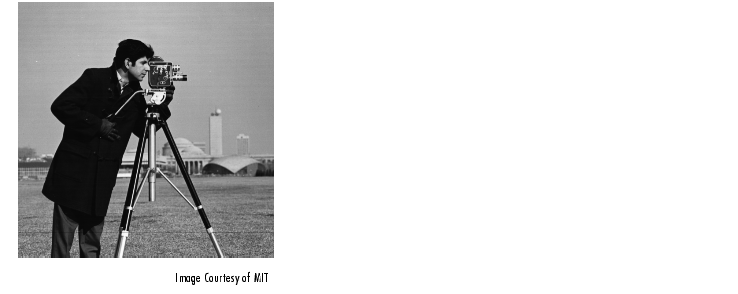```
2. Create the PSF.
• ```PSF = fspecial('motion',13,45);
figure; imshow(PSF,[],'notruesize'); title('Original PSF');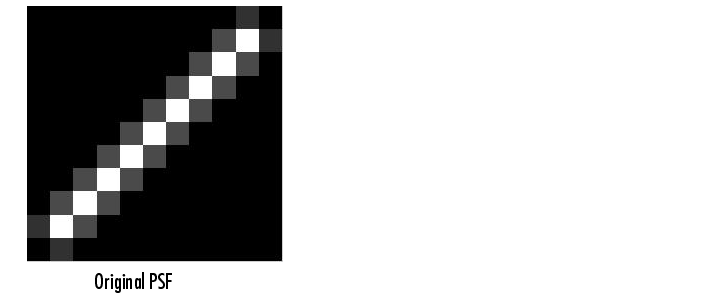```
3. Create a simulated blur in the image.
• ```Blurred = imfilter(I,PSF,'circ','conv');
figure; imshow(Blurred); title('Blurred Image');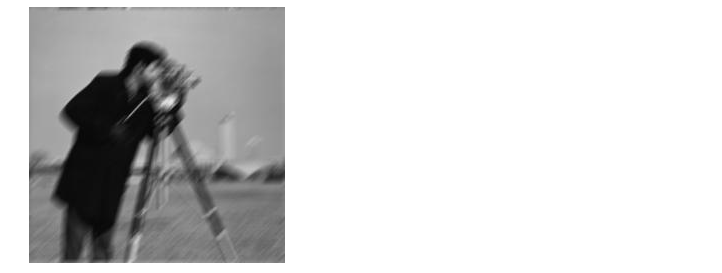```
4. Deblur the image, making an initial guess at the size of the PSF.
1. To determine the size of the PSF, examine the blurred image and measure the width of a blur (in pixels) around an obviously sharp object. In the sample blurred image, you can measure the blur near the contour of the man's sleeve. Because the size of the PSF is more important than the values it contains, you can typically specify an array of 1's as the initial PSF.

The following figure shows a restoration where the initial guess at the PSF is the same size as the PSF that caused the blur. In a real application, you might need to rerun `deconvblind`, experimenting with PSFs of different sizes, until you achieve a satisfactory result. The restored PSF returned by each deconvolution can also provide valuable hints at the optimal PSF size. See the Image Processing Toolbox deblurring demos for an example.

• ```INITPSF = ones(size(PSF));
[J P]= deconvblind(Blurred,INITPSF,30);
figure; imshow(J); title('Restored Image');
figure; imshow(P,[],'notruesize');
title('Restored PSF');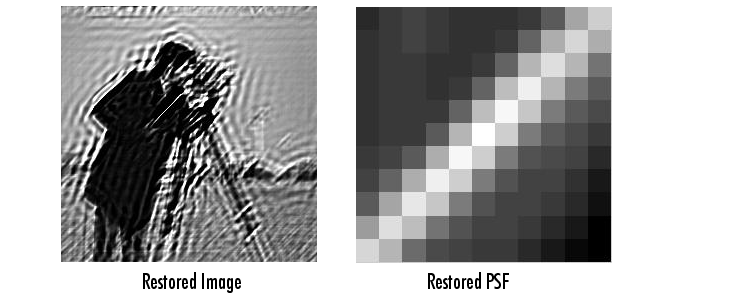```

Although `deconvblind` was able to deblur the image to a great extent, the ringing around the sharp intensity contrast areas in the restored image is unsatisfactory. (The example eliminated edge-related ringing by using the `'circular'` option with `imfilter` when creating the simulated blurred image in step 3.)

The next steps in the example repeat the deblurring process, attempting to achieve a better result by

• Eliminating high-contrast areas from the processing
• Specifying a better PSF
1. Create a `WEIGHT` array to exclude areas of high contrast from the deblurring operation. This can reduce contrast-related ringing in the result.
1. To exclude a pixel from processing, you create an array of the same size as the original image, and assign the value `0` to the pixels in the array that correspond to pixels in the original image that you want to exclude from processing. (See Accounting for Nonuniform Image Quality for information about `WEIGHT` arrays.)

To create a `WEIGHT` array, the example uses a combination of edge detection and morphological processing to detect high-contrast areas in the image. Because the blur in the image is linear, the example dilates the image twice. (For more information about using these functions, see Morphological Operations.) To exclude the image boundary pixels (a high-contrast area) from processing, the example uses `padarray` to assign the value 0 to all border pixels.

• ```WEIGHT = edge(I,'sobel',.28);
se1 = strel('disk',1);
se2 = strel('line',13,45);
WEIGHT = ~imdilate(WEIGHT,[se1 se2]);
figure; imshow(WEIGHT); title('Weight Array');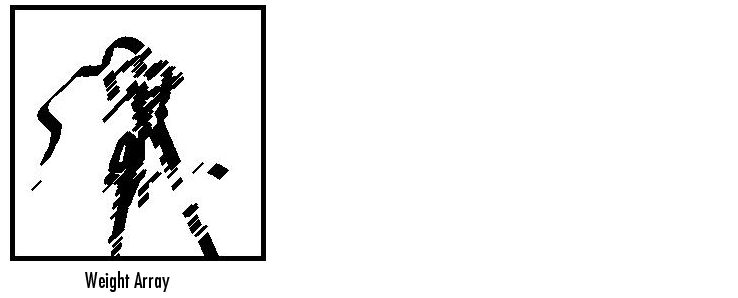```
1. Refine the guess at the PSF. The reconstructed PSF `P` returned by the first pass at deconvolution shows a clear linearity, as shown in the figure in step 4. For the second pass, the example uses a new PSF, `P1`, which is the same as the restored PSF but with the small amplitude pixels set to 0.
• ```P1 = P;
P1(find(P1 < 0.01))=0;
```
2. Rerun the deconvolution, specifying the `WEIGHT` array and the modified PSF. Note how the restored image has much less ringing around the sharp intensity contrast areas than the result of the first pass (step 4).
• ```[J2 P2] = deconvblind(Blurred,P1,50,[],WEIGHT);
figure; imshow(J2);
title('Newly Deblurred Image');
figure; imshow(P2,[],'notruesize');
title('Newly Reconstructed PSF');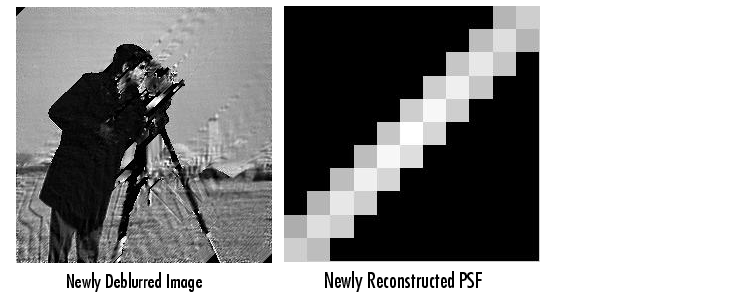```

Refining the Result

The `deconvblind` function, by default, performs multiple iterations of the deblurring process. You can stop the processing after a certain number of iterations to check the result, and then restart the iterations from the point where processing stopped. To use this feature, you must pass in both the blurred image and the PSF as cell arrays, for example, `{Blurred}` and `{INITPSF}`.

The `deconvblind` function returns the output image and the restored PSF as cell arrays. The output image cell array contains these four elements:

 Element Description `output{1}` Original input image `output{2}` Image produced by the last iteration `output{3}` Image produced by the next to last iteration `output{4}` Internal information used by `deconvlucy` to know where to restart the process

The PSF output cell array contains similar elements.

The `deconvblind` function supports several other optional arguments you can use to achieve the best possible result, such as specifying a damping parameter to handle additive noise in the blurred image. To see the impact of these optional arguments, as well as the functional option that allows you to place additional constraints on the PSF reconstruction, see the Image Processing Toolbox deblurring demos.Deblurring with the Lucy-Richardson Algorithm Creating Your Own Deblurring Functions